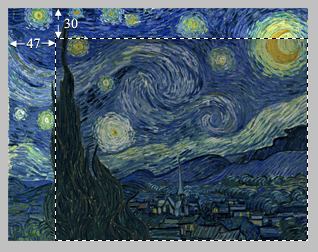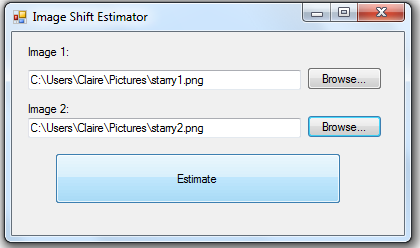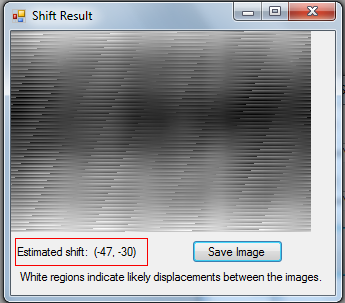This is a tool for estimating the amount of shift (displacement) between two images using FFT (Fast Fourier Transform). The images can be of different sizes. This method is faster than enumerating all the possible (x,y) shifts and selecting the right one, especially for large images. To perform the transforms it uses the fftw library.

More specifically it uses the 2D convolution function between the images. The steps for this are:
1. take the Fourier coefficients of the first image
2. take the same the second image,
3. find the element-wise product of the two results,
4. find the inverse Fourier transform of the above.

It shows the resulting image, with scaled colour channels so the darkest is black and the lightest is white. The light areas indicate likely amounts of shift. For example, a light area at co-ordinate (5, 6) means the second image was probably shifted 5 pixels across, 6 units down from the first.

As an example of using it, consider cropping an image so that the resulting image is 47 units across and 30 units down.Point the program toward the two image files and hit ‘Estimate’.The dialog will (quickly) show a visual result of the convolution, and the estimated shift. The shift matches with where the image was cropped. The units are the position of the first image, in pixels, relative to the second one.

A brute-force implementation of the same thing is very doable but takes around 30 seconds on this sample, so it’s a pretty significant speedup!The source code is compiled using Visual Studio 2008. There are three project files. ConvolveShift is the main executable, a Windows Forms program in C#. Native is a C++ DLL that does most of the real work. NativeDriver is a non-graphical program for testing Native.

September 13th, 2012 at 5:00 pm | Comments & Trackbacks (0) | Permalink